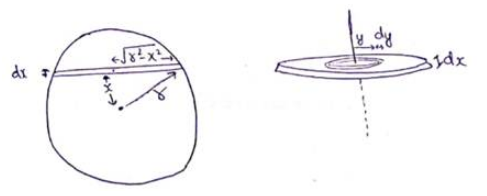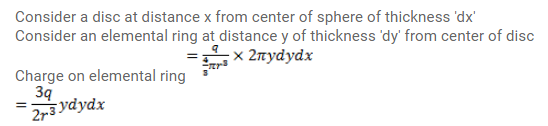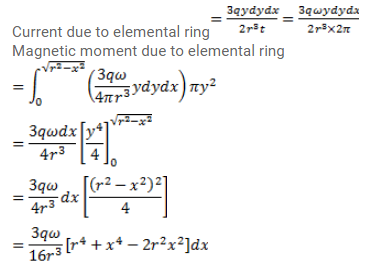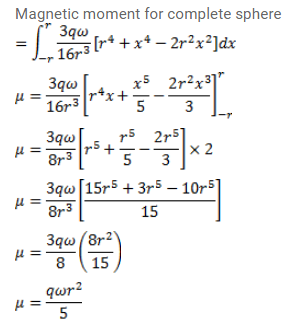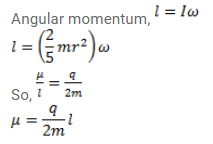# Consider a solid sphere of radius r and mass m

Question:

Consider a solid sphere of radius $\mathrm{r}$ and mass $\mathrm{m}$ which has a charge $\mathrm{q}$ distributed uniformly over its volume. The sphere is rotated about a diameter with an angular speed $\omega$. Show that the magnetic moment $\mu$ and the angular momentum I of the sphere are related as $\mu=\frac{q}{2 m} l$.

Solution: